Example Questions

Example Question #21 : How To Use Foil With The Distributive Property

Find the product of the two binomials: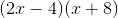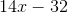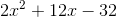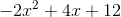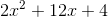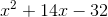Explanation:

Use FOIL (First Outside Inside Last) to multiply the binomials: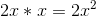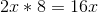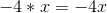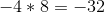now combine like terms and you get the result:Example Question #22 : How To Use Foil With The Distributive Property

Expand the following: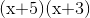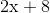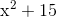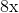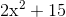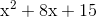Explanation:

To reach the solution, use the FOIL method of the distributive property. This stands for First, Outer, Inner, Last. In this particular problem, we begin by multiplying the first two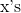together, yielding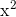. Then, we multiply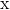by the outer number, which is, yielding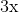. Next, we multiply the two inner numbers,and, yielding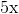. Finally, we multiply the last two numbers,and, yielding. Thus, when we add all those together, the answer is.

Example Question #23 : How To Use Foil With The Distributive Property

Distribute: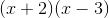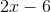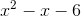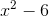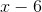Explanation:

To FOIL using the distributive property, multiply the first terms together, then the outer terms, then the inner terms, and finally, the last terms.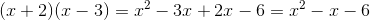Example Question #24 : How To Use Foil With The Distributive Property

Distribute: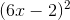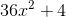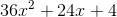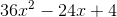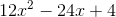Explanation:

To FOIL using the distributive property, multiply the first terms together, then the outer terms, then the inner terms, and finally, the last terms.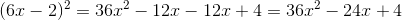Example Question #21 : Distributive Property

Distribute: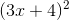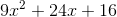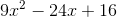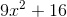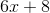Explanation:

FOIL using the distributive property: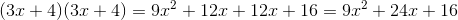Example Question #26 : How To Use Foil With The Distributive Property

Distribute: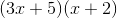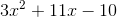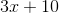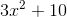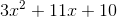Explanation:

FOIL using the distributive property: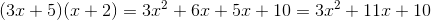Example Question #27 : How To Use Foil With The Distributive Property

Distribute: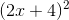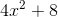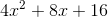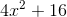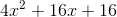Explanation:

FOIL using the distributive property.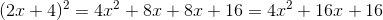Example Question #28 : How To Use Foil With The Distributive Property

Distribute: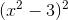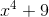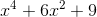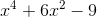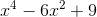Explanation:

FOIL using the distributive property.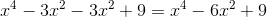Example Question #29 : How To Use Foil With The Distributive Property

Distribute: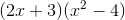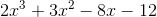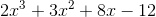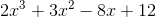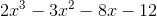Explanation:

FOIL using the distributive property.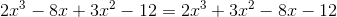Example Question #30 : How To Use Foil With The Distributive Property

Distribute: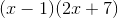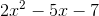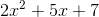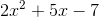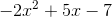Explanation:

Use the distributive property to FOIL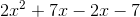Simplify.